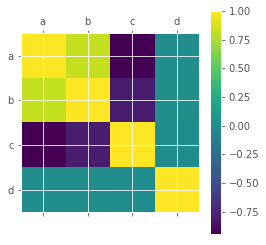## Correlation in Python

• Post author:
• Post category:Python

# Correlation in Python

Correlation values range between -1 and 1.

There are two key components of a correlation value:

• magnitude – The larger the magnitude (closer to 1 or -1), the stronger the correlation
• sign – If negative, there is an inverse correlation. If positive, there is a regular correlation.

### Positive Correlation

Let’s take a look at a positive correlation. Numpy implements a corrcoef() function that returns a matrix of correlations of x with x, x with y, y with x and y with y. We’re interested in the values of correlation of x with y (so position (1, 0) or (0, 1)).

In :
import numpy as np

np.random.seed(1)

# 1000 random integers between 0 and 50
x = np.random.randint(0, 50, 1000)

# Positive Correlation with some noise
y = x + np.random.normal(0, 10, 1000)

np.corrcoef(x, y)

Out:
array([[ 1.        ,  0.81543901],
[ 0.81543901,  1.        ]])

This correlation is 0.815, a strong positive correlation, let’s take a look at a scatter chart.

In :
import matplotlib
import matplotlib.pyplot as plt
%matplotlib inline
matplotlib.style.use('ggplot')

plt.scatter(x, y)
plt.show()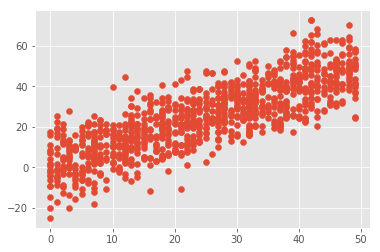### Negative Correlation

What happens to our correlation figure if we invert the correlation such that an increase in x results in a decrease in y?

In :
# 1000 random integers between 0 and 50
x = np.random.randint(0, 50, 1000)

# Negative Correlation with some noise
y = 100 - x + np.random.normal(0, 5, 1000)

np.corrcoef(x, y)

Out:
array([[ 1.        , -0.94957116],
[-0.94957116,  1.        ]])

Our correlation is now negative and close to 1. Let’s take a look at what this looks like graphically:

In :
plt.scatter(x, y)
plt.show()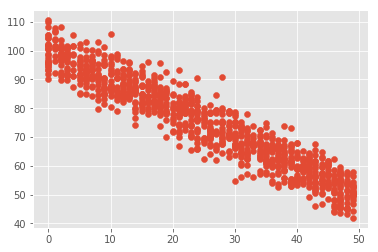### No/Weak Correlatio

What if there is no correlation between x and y?

In :
x = np.random.randint(0, 50, 1000)
y = np.random.randint(0, 50, 1000)

np.corrcoef(x, y)

Out:
array([[ 1.        , -0.00554681],
[-0.00554681,  1.        ]])

Here we see a very small value for the correlation between x and y, indicating no correlation.

Again, let’s plot this and take a look, we see there is no correlation between x and y:

In :
plt.scatter(x, y)
plt.show()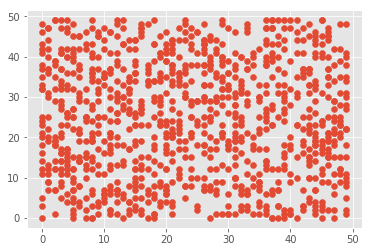### Correlation Matrix

If we’re using pandas we can create a correlation matrix to view the correlations between different variables in a dataframe:

In :
import pandas as pd

df = pd.DataFrame({'a': np.random.randint(0, 50, 1000)})
df['b'] = df['a'] + np.random.normal(0, 10, 1000) # positively correlated with 'a'
df['c'] = 100 - df['a'] + np.random.normal(0, 5, 1000) # negatively correlated with 'a'
df['d'] = np.random.randint(0, 50, 1000) # not correlated with 'a'

df.corr()

Out:
a b c d
a 1.000000 0.825361 -0.948845 0.009802
b 0.825361 1.000000 -0.789391 0.011852
c -0.948845 -0.789391 1.000000 -0.003228
d 0.009802 0.011852 -0.003228 1.000000

We can also view these correlations graphically as a scatter matrix:

In :
pd.scatter_matrix(df, figsize=(6, 6))
plt.show()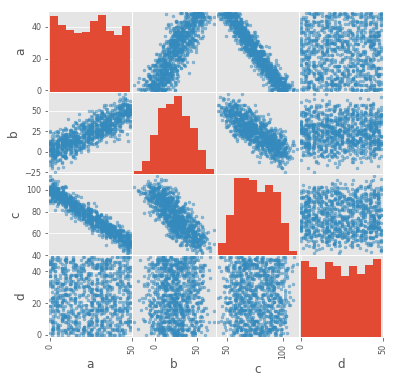Or we can directly plot a correlation matrix plot:

In :
plt.matshow(df.corr())
plt.xticks(range(len(df.columns)), df.columns)
plt.yticks(range(len(df.columns)), df.columns)
plt.colorbar()
plt.show()